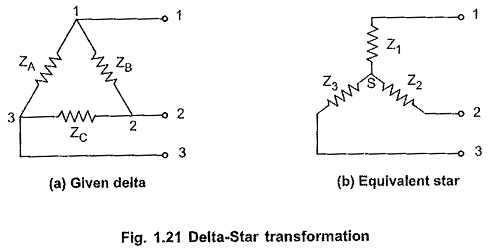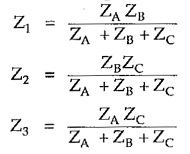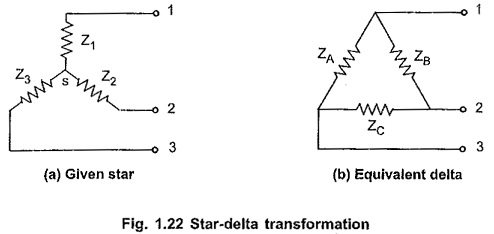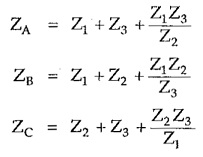## Delta to Star Conversion and Star to Delta Conversion:

In the complicated networks involving large number of elements, Delta to Star and Star to Delta Conversion considerably reduce the complexity of the network and network can be analysed very quickly. These transformations allow us to replace three star connected elements of network, by equivalent Delta connected elements without affecting currents in other branches and vice versa.

#### Delta to Star Transformation Formula:

Consider three impedances ZA , ZB and ZC connected in delta as shown in the Fig. 1.21(a). The equivalent star is shown in the Fig. 1.21(b).The formulae to calculate equivalent star from the given delta are,#### Star Delta Transformation Formula:

Consider three impedances Z1, Z2 and Z3 connected in star as shown in the Fig. 1.22(a). The equivalent delta is shown in the Fig. 1.22(b).The formulae to calculate equivalent delta from the given star are,The delta star transformation is also called π – T transformation while star delta transformation is also called T – π transformation.

Scroll to Top